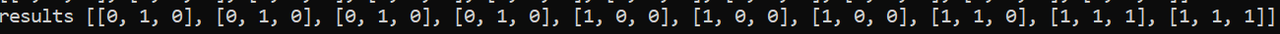Counting Number of Element in a smart way - Printable Version +- Python Forum (https://python-forum.io) +-- Forum: Python Coding (https://python-forum.io/forum-7.html) +--- Forum: General Coding Help (https://python-forum.io/forum-8.html) +--- Thread: Counting Number of Element in a smart way (/thread-30847.html) Counting Number of Element in a smart way - quest - Nov-09-2020 Hello, I have an arrayI want to count the elements in the array and I can count the number of elements with this code ```a0 = sum(np.all((results-np.array([0,0,0]))==0, axis=1)) x1 = sum(np.all((results-np.array([0,0,1]))==0, axis=1)) x2 = sum(np.all((results-np.array([0,1,0]))==0, axis=1)) x3 = sum(np.all((results-np.array([0,1,1]))==0, axis=1)) x4 = sum(np.all((results-np.array([1,0,0]))==0, axis=1)) x5 = sum(np.all((results-np.array([1,0,1]))==0, axis=1)) x6 = sum(np.all((results-np.array([1,1,0]))==0, axis=1)) x7 = sum(np.all((results-np.array([1,1,1]))==0, axis=1))```But I want to do it in a shorter way. I don't want to specify my all triplets. This time I have 8 different elements so I could write it by hand but if I have 100 different triplets, I couldn't use this code Is there any nicer way to do it? Bests RE: Counting Number of Element in a smart way - bowlofred - Nov-09-2020 If the only objection is writing out the triplets, you could do the following. It runs the same commands, just does it in a loop. ```from itertool import product triplets = tuple(product((0, 1), repeat = 3)) sums = {} for triple in triplets: sums[triple] = sum(np.all((results-np.array(triple))==0, axis=1))```The sums will be in a dict `sums` rather than as separate variables. But you can pull them out, or you could change how the keys are named. However, this doesn't change the fundamental calls made. Perhaps someone else can see if there's a better way to have numpy do the sum more directly. RE: Counting Number of Element in a smart way - quest - Nov-09-2020 Thank you very much !